Home » Handbook of O Level Physics » UNIT 15: CONVERGING AND DIVERGING LENSES

# UNIT 15: CONVERGING AND DIVERGING LENSES

• Converging lenses: They are fatter at the center, e.g., biconvex lens, plano-convex lens, etc.

• Diverging lenses: They are thinner at the center, e.g., biconcave lens, plano-concave lens, etc.

• Principal focus (or focal point): It is the point where all rays close to and parallel to the principal axis converge after refraction from a converging lens.

• Focal length: Focal length is the distance between the optical center of the lens and the principal focus.

• Important rays for converging lens are:

1. An incident ray that passes straight through optical centre.

2. An incident ray parallel to principal axis is refracted to pass through principal focus (F).

3. An incident ray passing through principal focus (F) is refracted to become parallel to principal axis.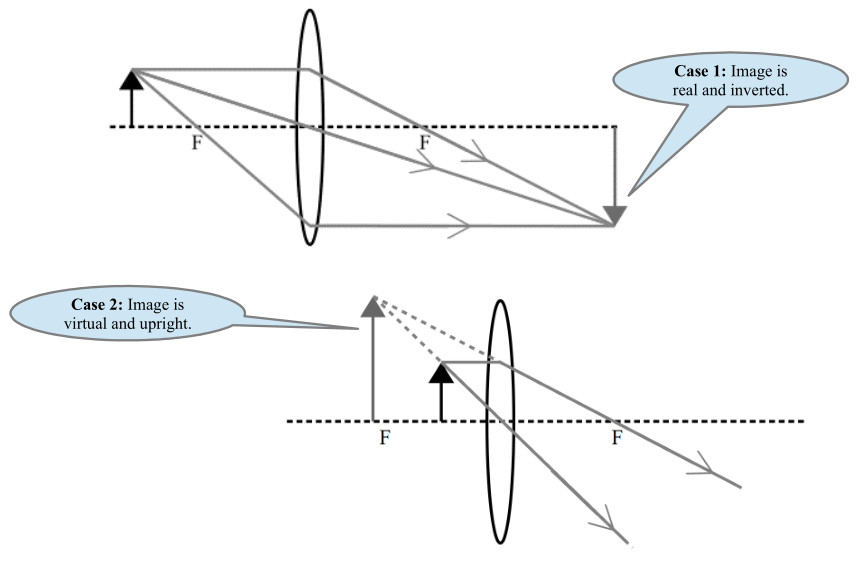• Important rays for diverging lens are:

1. An incident ray that passes straight through optical centre.

2. An incident ray parallel to principal axis is refracted such that it appears to be coming from the principal focus (F) on the side of the object.

3. An incident ray aiming for principal focus (F) on far side is refracted to become parallel to principal axis.• Human eye

• Parts and their functions:

• a crystalline biconvex lens to focus light

• a retina having light sensitive cells acts as a screen

• ciliary muscles to change the curvature of the lens

• an iris controls the amount of light entering the eye

• Working: The human eye can change the lens curvature (and hence the principal focus) to view objects that are far or near.

• Shortsightedness (Myopia): The eye can focus light from near objects but not from objects that are far.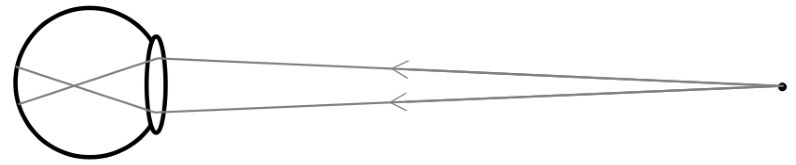• It is corrected like this.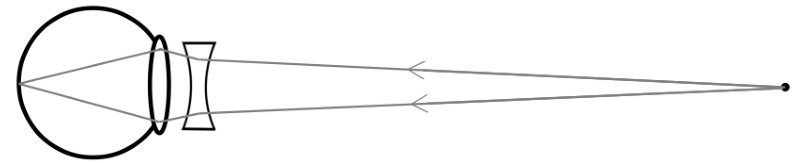• Farsightedness (Hyperopia): The eye can focus light from far objects but not from those that are near.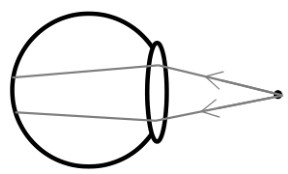• It is corrected like this.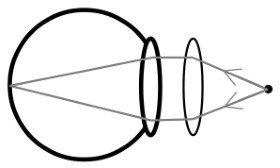• Linear magnification (m)

m = (image height) / (object height)

m = (image distance) / (object distance)

Note: “m = 1” means image is same size as the object. “m = 2” means image is twice the size of the object and so on…

• Properties of an image

• inverted / upright

• real / virtual

• magnified / diminished / same size

• greater / same / less distance from lens compared to the object

• Virtual images are always upright and real images are always inverted.

• Lenses are used in cameras, projectors, photocopiers, telescopes, etc.